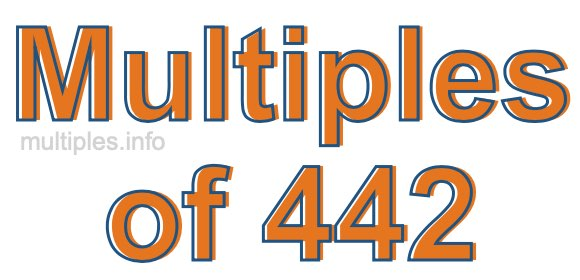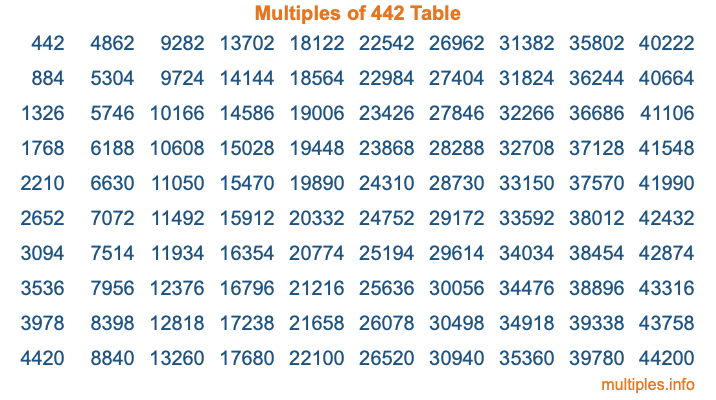Multiples of 442Welcome to the Multiples of 442 page. Here we will first teach you everything you will ever need to know about the multiples of 442, and then give you a study guide summary of everything we taught you to make sure you remember it all. Use this page to look up facts and learn information about the multiples of 442. This page will make you a multiples of four hundred forty-two expert!

Definition of Multiples of 442
Multiples of 442 are all the numbers that when divided by 442 equal an integer. Each of the multiples of 442 are called a multiple. A multiple of 442 is created by multiplying 442 by an integer.

Therefore, to create a list of multiples of 442, you start with 1 multiplied by 442, then 2 multiplied by 442, then 3 multiplied by 442, and so on for as long as you want. Thus, the list of the first five multiples of 442 is 442, 884, 1326, 1768, and 2210. To see a larger list of multiples of 442, see the printable image of Multiples of 442 further down on this page. We also have a category where you can choose any nth multiple of 442.

Multiples of 442 Checker
The Multiples of 442 Checker below checks to see if any number of your choice is a multiple of 442. In other words, it checks to see if there is any number (integer) that when multiplied by 442 will equal your number. To do that, we divide your number by 442. If the the quotient is an integer, then your number is a multiple of 442.

Is  a multiple of 442?

Least Common Multiple of 442 and ...
A Least Common Multiple (LCM) is the lowest multiple that two or more numbers have in common. This is also called the smallest common multiple or lowest common multiple and is useful to know when you are adding our subtracting fractions. Enter one or more numbers below (442 is already entered) to find the LCM.

Check out our LCM Calculator if you need more details about the Least Common Multiple or if you need the LCM for different numbers for adding and subtraction fractions.

nth Multiple of 442
As we stated above, 442 is the first multiple of 442, 884 is the second multiple of 442, 1326 is the third multiple of 442, and so on. Enter a number below to find the nth multiple of 442.

th multiple of 442

Multiples of 442 vs Factors of 442
442 is a multiple of 442 and a factor of 442, but that is where the similarities end. All postive multiples of 442 are 442 or greater than 442. All positive factors of 442 are 442 or less than 442.

Below is the beginning list of multiples of 442 and the factors of 442 so you can compare:

Multiples of 442: 442, 884, 1326, 1768, 2210, etc.

Factors of 442: 1, 2, 13, 17, 26, 34, 221, 442

As you can see, the multiples of 442 are all the numbers that you can divide by 442 to get a whole number. The factors of 442, on the other hand, are all the whole numbers that you can multiply by another whole number to get 442.

It's also interesting to note that if a number (x) is a factor of 442, then 442 will also be a multiple of that number (x).

Multiples of 442 vs Divisors of 442
The divisors of 442 are all the integers that 442 can be divided by evenly. Below is a list of the divisors of 442.

Divisors of 442: 1, 2, 13, 17, 26, 34, 221, 442

The interesting thing to note here is that if you take any multiple of 442 and divide it by a divisor of 442, you will see that the quotient is an integer.

Multiples of 442 Table
Below is an image of the first 100 multiples of 442 in a table. The table is in chronological order, column by column. The first column has the first ten multiples of 442, the second column has the next ten multiples of 442, and so on.The Multiples of 442 Table is also referred to as the 442 Times Table or Times Table of 442. You are welcome to print out our table for your studies.

Negative Multiples of 442
Although not often discussed or needed in math, it is worth mentioning that you can make a list of negative multiples of 442 by multiplying 442 by -1, then by -2, then by -3, and so on, to get the following list of negative multiples of 442:

-442, -884, -1326, -1768, -2210, etc.

Multiples of 442 Summary
Below is a summary of important Multiples of 442 facts that we have discussed on this page. To retain the knowledge on this page, we recommend that you read through the summary and explain to yourself or a study partner why they hold true.

There are an infinite number of multiples of 442.

A multiple of 442 divided by 442 will equal a whole number.

442 divided by a factor of 442 equals a divisor of 442.

The nth multiple of 442 is n times 442.

The largest factor of 442 is equal to the first positive multiple of 442.

442 is a multiple of every factor of 442.

442 is a multiple of 442.

A multiple of 442 divided by a divisor of 442 equals an integer.

442 divided by a divisor of 442 equals a factor of 442.

Any integer times 442 will equal a multiple of 442.

Multiples of a Number
Here you can get the multiples of another number, all with the same attention to detail as we did for multiples of 442 on this page.

Multiples of
Multiples of 443
Did you find our page about multiples of four hundred forty-two educational? Do you want more knowledge? Check out the multiples of the next number on our list!